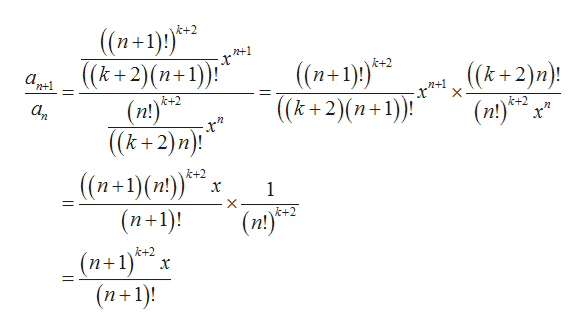# If k is a positive integer, find the radius of convergence, R, of the seriesΣ(n!)k+2-xn((k2)n)!n 0R =

Question
check_circle

Step 1

It is required to calculate the radius of convergence R of the series:

Step 2

Here,

Step 3

Now, find the ratio for...help_outlineImage Transcriptionclose(n+1)!)** ((k+2)(n+1) k+2 ((n+1)* ((k+2)(n+1)) ! (k+2)n)! (n)o n+1 k+2 (п!) а, k+2 ((k+2)n)! (n+1)(n) (n+1)! k+2 x k+2 (nt)3 (n+1)*x (n+1)! k+2 fullscreen

### Want to see the full answer?

See Solution

#### Want to see this answer and more?

Solutions are written by subject experts who are available 24/7. Questions are typically answered within 1 hour.*

See Solution
*Response times may vary by subject and question.
Tagged in

### Math